Viewing posts from February, 2016

## Where are the Aliens :)

This subject has a curious history.  It never states the obvious.  Most likely any encounter with an Alien Civilization would take place through their Robotic Explorers - not unlike our own case.   If there were any life form in Mars or Europa - they would had meet our robotic devices.

# The "belief' of the importance or relevance of a placebo effect always bothered me in the same way as the power of prayer.

I've never worked on clinical trials statistics so I was never privy to data from double-blind randomized controlled trials (RCT).  Someone in the DC's Improbable Science did a great article on the subject.

## The Riemann Hypothesis and the Hypergeometrical Universe - ReviewRiemann Hypothesis and The Hypergeometrical Universe

We are jump starting this blog to finish up the calculations of the hyperon family and isotopes.
This is reproduced here just to jog your memory...:)Our love for knowledge and beauty has always driven us to find the most sublime analogies to represent what we are seeing in the Universe. Einstein wanted to read God's Mind. The Hypothetical Higgs Boson has been named by some as the God Particle. I equated the steps of my Lightspeed Expanding Hyperspherical Universe as being somewhat similar to the Pendulum of Brahma. At each cycle we see ourselves changed by that Universal Operator.
Mathematics is always the Golden Standard of beauty. We always hope to find a nicely fitting mathematical equation to describe the whole Universe. Mathematicians (String Theorists) are especially hopeful..:). Of course, after all, that would be redemption to their endeavor of creating the least physical (most mathematical) theory in history.
Euler's Beta Function relationship to the Strong Force model was immediately recognized as the sign we are on the right track, after all, for a theory to be correct it has to be aesthetically beautiful….:) Form over content…very typical of our Mankind always driven by superficial calculations….:)

It just happens that there isn't any indication that physics doesn't matter, that is, there isn't any indication that at the end of all, we will have everything described by a simple mathematical equations that overrules all physical properties.
For example, there is the wave equation which describes all waves as long as they have a natural velocity or one knows the elasticity of the medium where those waves propagate. This means that even though we have the knowledge of an equation which would describe such a general phenomena as waves, the physics is still there in the form of a pesky constant.. :)
This does not demerit the scientists who found those beautiful equations, it just serves as a reminder that there might be a limit on what mathematical abstraction can achieve.
It has been said with some understated deepness that the Riemann Hypothesis might be the solution to the Theory of Everything, that is, something, some force, some string might be represented somehow by the zeros of the Zeta function along the critical line. The details are fuzzy since this is just a wild (albeit educated..:)  guess...:)
Scientists look down to Horoscopists all their lives…:)  It is well know that vague statements can always find resonance within some fraction of the population and thus keep the readers happy and aware of their daily best options…:)

One might say that the same happens in Science. There is a difference. Since we are a much more educated bunch than the average person, our guesses are better educated..:) that is, there is something deep in the number theory applied to Primes..:)
Of course, this is just because people doesn't know how to think about numbers and one should expect that if the zeros of an equation are related to primes, most likely the logic behind the construction of the equation is such that primes will be part of the equation zeros..:)
It is like being surprised that f(x)=(x-1)*(x-2) has zeros equal to 1 and 2.

Number theory is just not developed enough to see the underlying logic behind the Riemann Hypothesis and the Zeta Function (or Eta Function on the Analytic Continuation).

As usual, the statement that the Riemann Hypothesis should be relevant to our understanding of the Universe can be decomposed into the simpler statement that Primes will be relevant..:) I am a simple minded man, so I will cut to the chase and explore this proposition..:)
Nobody bothered to think about Primes because nothing in Physics looks like Primes. If a poor string theorist looks around and think about making Primes to be some property of strings them we might end up with 10^500000000000000 possible Universes with different string theories explaining each one of them..:)
A Standard Model Physicist is not in much better position. Let say that zero are electromagnetic waves, the ONE is…J The next basic particles are electron and Proton … Neutron might be TWO but that does not solve the problem. Electrons and Protons are certainly not equal in the Standard Model and the analogy dies there…:)

Of course, that is not the case in the Hypergeometrical Universe!!!! :)

ARE FERMION PARTICLES PRIMES
YES.  I decided to add ONE to the prime sequence just as a rebellious guy I am..:)
In my theory one can easily relate ZERO to the dilaton field and its spatial modulation (electromagnetic waves).

ONE is clearly the Fundamental Dilator which represents all four fundamental particles (Electron, Proton, AntiElectron, AntiProton).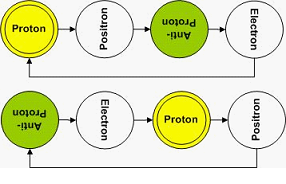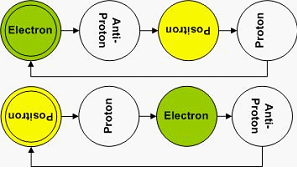TWO is of course the Neutron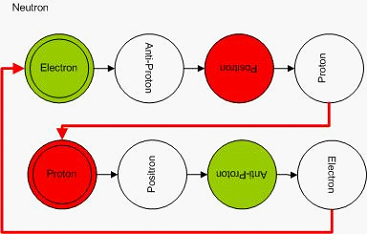THREE are the Pions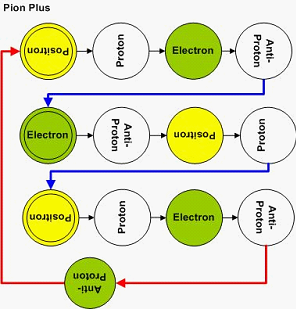FIVE are the CRAZY DELTASSEVEN are the KAONS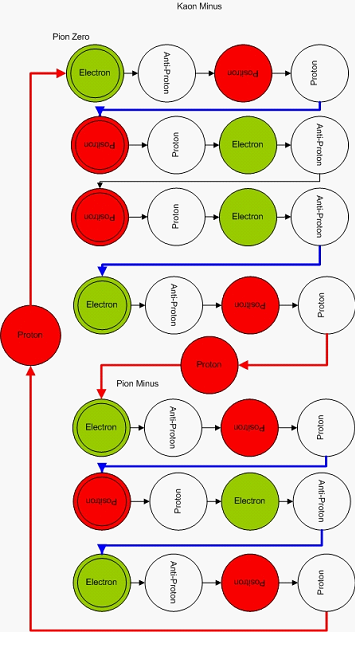ELEVEN are the XIS Star – This Channel decays into a KaonZero and a Pion Minus.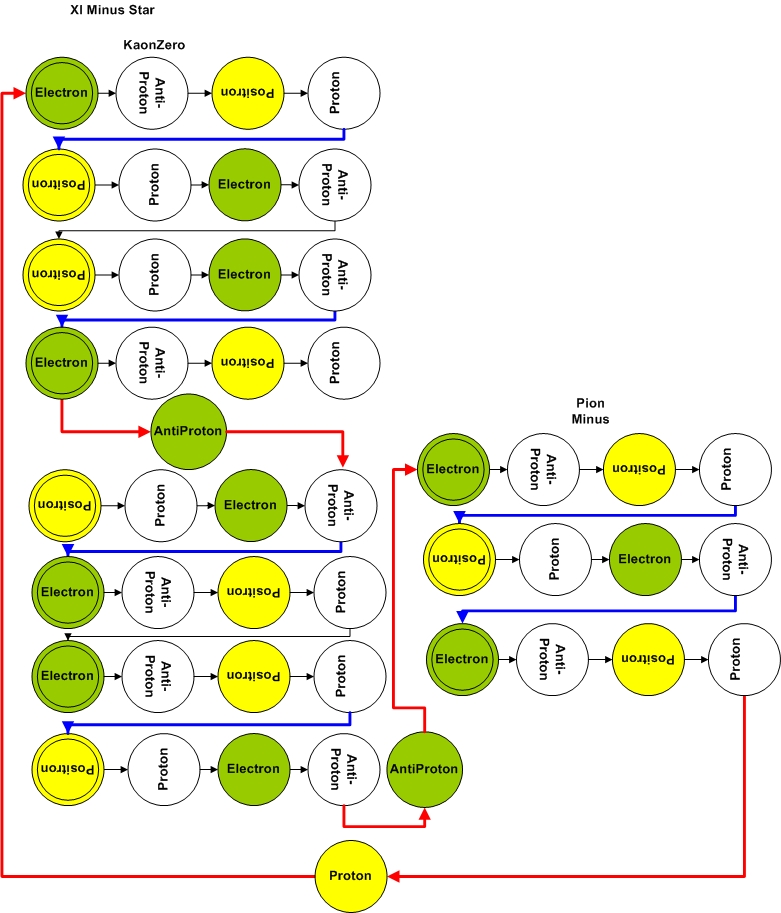THIRTEEN are the LUCKY OMEGAS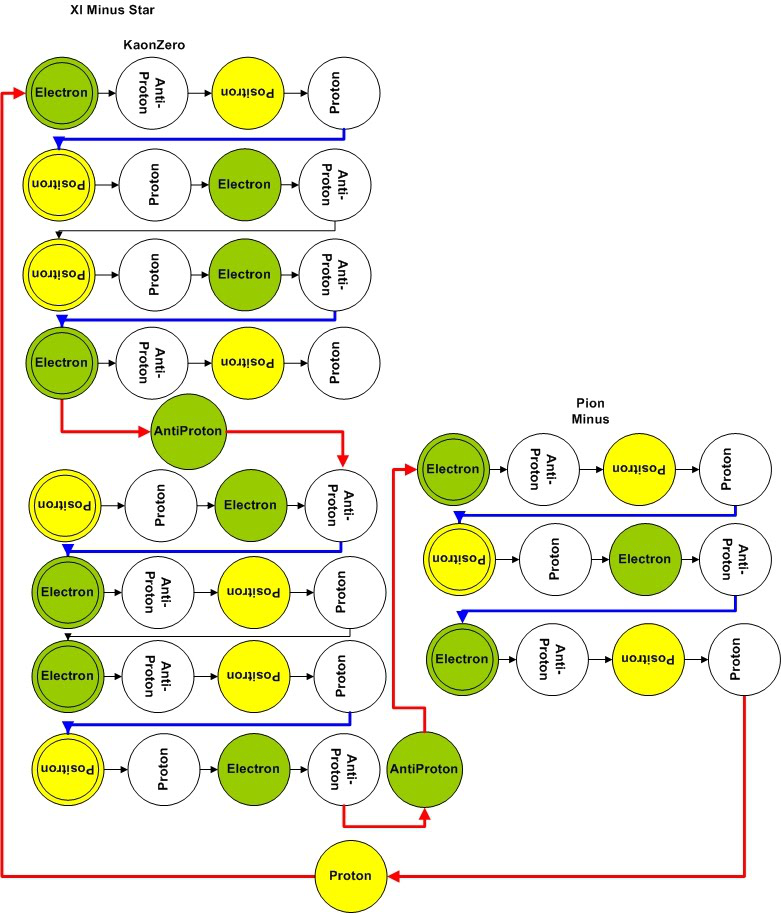ARE Neutral Majorama PARTICLES 2^N NUMBERS

YES. I am sure the numerologists will have a field day..:)

As in any good Horoscope, there is a caveat. There are exclusions to the Prime Rule. They are associated with another nice mathematical formula, the formula for 2 to the power of another number. (2,4,8,16…)

TWO is the precursor to a Gamma photon from the annihilation of a Positron-Electron pair.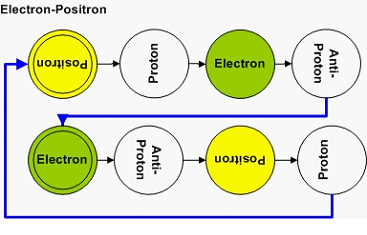FOUR is the Pion ZeroEIGTH is the KAON ZEROLater, I will show how the mathematical space topology and mathematical instrumentation needed to solve the Riemann Hypothesis also describes the mass of the Hyperons…:)

A hint. Look at the Fundamental Dilator coherence 'energy' diagram. The degenerated states on the two potential wells lose their degeneration due to the finite velocity of light, which creates a time delay for a spatial rotation within the 3D Lightspeed Expanding Hypersphere.

Think about what does it means to be real…:) and read the Meaning of Material Existence blog I wrote in the past.ARE THERE Neutral PARTICLES = 2*N NUMBERS

As in any good Horoscope, there is always something for everyone. It just happens that 2N is also a valid mapping for Neutral Particles.

Of course, since they decompose into opposite charged particles, they have to be the SUM OF TWO PRIMES (Goldbach's Conjecture) or 2 Majorama Particles…:)

SIX is the DELTA ZERONow that you know the answer to the question, it should be easier to derive the equation that describes the process...:) I wrote a book called The Flying Orchestra, which represents our journey through the Universe inside the Ligthspeed Expanding Hyperspherical Universe. Most of us :) are composed of isotopes, which turned out to be quite harmonious dimensional notes. The Hyperons are the basic notes of our Universe, that is, the most unique, cacophonous notes one can create- like Heavy Metal...:)

In comparison, the isotopes are like Mozart Symphonies...NAH....in fact, they are quite boring and more like Kenny G

In the next few blogs, I will address the isotopes, the mass equation (or God Equation if you so prefer :) .

I am awaiting for Steven Chu to say something before I write about how to make a neutral particle accelerator or anything accelerator or dimensional accelerator...:)

Cheers,

MP